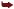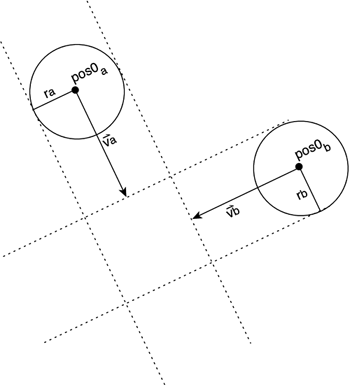# Moving Tests

Imagine a game of snooker with balls moving around quickly. If we need to compute the interactions between the different balls efficiently and with precision, we cannot consider them to be static objects. We need to compute trajectories, making sure we detect both if a collision took place and when it took place. This is a relatively young field and only recent advances in real-time physics have made such computations possible.

#### Sphere: Sphere

We will now derive a test for moving spheres, which is very useful for moving objects. I will use it as an example of how to solve algebraic, restriction-based equations so we can compute collisions. You can adapt the following case for other shapes as well.

To begin with, here are the two expressions for two points moving in space. Each point is determined by its position when time=0 and its velocity vector:

` posa=pos0a + va*t posb=pos0b + vb*t `

Now, we can blend the two equations by computing the difference between them, that is, the vectorial difference between both positions:

` posa-posb = dpos = (pos0b-pos0a)+(vb-va)*t = dpos0 + dv*t `

Now, let's square the equation:

` dpos2 = dpos0 + 2*dpos0*dv*t + dv2*t2 `

Expanding it to incorporate the X, Y, and Z terms, we get the following expression for the distance between the two sphere centers:

` dist = sqrt       (dpos0.x2 + dpos0.y2 + dpos0.z2 + 2*dpos0.x*dv.x*t + 2*dpos0.y*dv.y*t + 2*dpos0.z*dv.z*t + dv.x2*t2 + dv.y2*t2 + dv.z2*t2) `

Figure 22.7 illustrates the situation described by the preceding equation. By grouping terms together, we reach the following expression:

` dist = sqrt ( A + B*t + C*t2) `

where

` A = dpos.x2 + dpos.y2 + dpos.z2 = |dpos|2 B = 2*(dpos.x*dv.x + dpos.y*dv.y + dpos.z*dv.z) = 2*(dpos dot dv) C = dv.x2 + dv.y2 + dv.z2= |dv|2 `
##### Figure 22.7. Moving sphere test.If we forget about dist, and square both sides of the equation to compute dist squared instead, we get

` dist2 = A + B*t + C*t2 `

Now, let's incorporate the radii to check for a collision. A collision happens if, for any moment in time

` dist = r1 + r2 `

where dist is the distance we were just working on, and r1/r2 are the radii. Now, let's square the equation:

` dist2 = (r1+r2)2 `

This can now be blended with our earlier equation to produce

` (r1+r2)2 = A + B*t + C*t2 `

By rearranging terms so this equation fits with our quadratic solving formulas from school, we get

` C*t2 + B*t + A-(r1+r2)2 = 0 `

or

` C*t2 + B*t + A = 0 `

Assuming a change in the value of A, which right now is

` A = dpos.x2 + dpos.y2 + dpos.z2 = |dpos|2   (r1+r2)2 `

The equation is solved by

` t = -B +/- sqrt (B2   4AC) / 2A `

So there is a collision if

` B2 > 4AC `

Then, we assume

` B2= (2*(dpos dot dv))2 = 4*(dpos dot dv)2 4AC= 4* (|dpos|2   (r1+r2)2) *  |dv|2 `

The solution is that the collision occurs if the following is true:

` 4*(dpos dot dv)2  >  4* (|dpos|2   (r1+r2)2) *  |dv|2 `

or, for elegance:

` (dpos dot dv)2  >  (|dpos|2   (r1+r2)2) *  |dv|2 `Core Techniques and Algorithms in Game Programming2003
ISBN: N/A
EAN: N/A
Year: 2004
Pages: 261